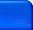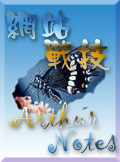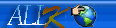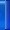搜尋    會員登入回首頁 特別收藏 寫信給亞瑟 常用連結網站 好文章分享 亞瑟相簿 好書收藏 珍藏影片 心情日記 星座探索解析 亞瑟留言版亞瑟心世界META 是什麼??附錄-A-3 META 是什麼??

<META HTTP-EQUIV=\"refresh\" CONTENT=\"15\">

<A HREF=\"javascript:history.go(0)\">重新下載</A>

<INPUT TYPE=\"button\" onClick=\"history.go(0)\" VALUE=\"重新下載\">

<META HTTP-EQUIV=\"refresh\" CONTENT=\"3;URL=http://www.geocities.com/alfredcave/index.html\">

META與搜尋引擎

<META NAME=\"keywords\" CONTENT=\"key,word,keyword,關鍵字\">

<META NAME=\"description\" CONTENT=\"好棒的網頁啊！\">

<META NAME=\"author\" CONTENT=\"艾爾弗烈德\">

<META NAME=\"expires\" CONTENT=\"31 December 2002\">

<META NAME=\"distribution\" CONTENT=\"global\">

<META NAME=\"revisit-after\" CONTENT=\"30 days\">

<META NAME=\"robots\" CONTENT=\"all\">

<META NAME=\"expires\" CONTENT=\"-1\"> 或
<META NAME=\"pragma\" CONTENT=\"no-cache\"> (僅IE適用)